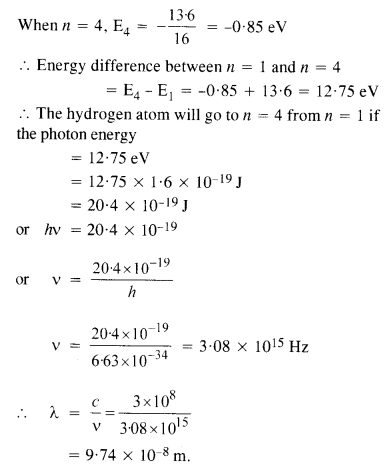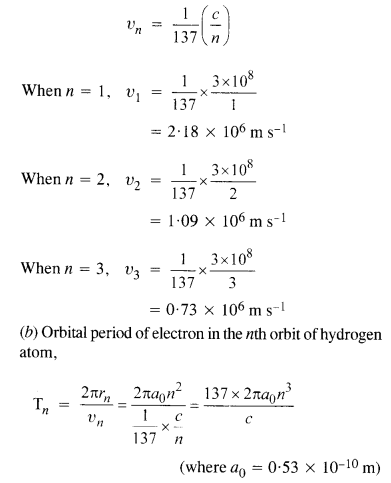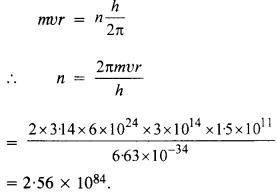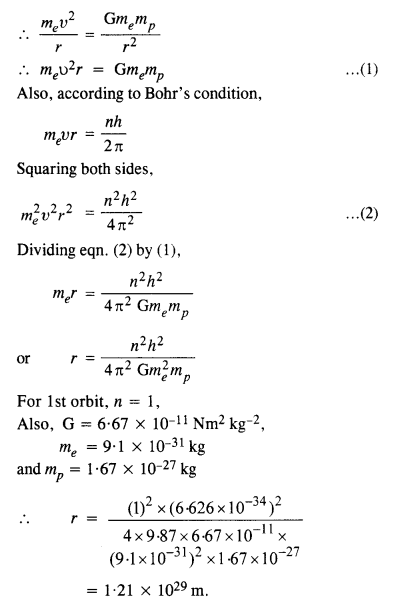# NCERT Solutions for Chapter 12 Atoms

Question 1.

Choose the correct alternative from clues given at end of each statement:

1. The size of the atom in Thomson’s model is the atomic size in Rutherford’s model.(much greater than/no different from/much less than.)
2. In the ground state of…………… electrons are in stable equilibrium, while in ……………..  electrons always experience a net force. (Thomson’s model/Rutherford’s model.)
3. A classical atom based on…………. is doomed to collapse. (Thomson’s model/Rutherford’s model.)
4. An atom has a nearly continuous mass distribution in a………….. but has a highly non­uniform mass distribution in (Thomson’s model/Rutherford’s model.)
5. The positively charged part of the atom possesses most of the mass in…………. (Rutherford’s model/both the models.)

1. no different from
2. Thomson’s model; Rutherford’s model.
3. Rutherford’s model.
4. Thomson’s model, Rutherford’s model.
5. both the models.

Question 2.

Suppose you are given a chance to repeat the alpha-particle scattering experiment using a thin sheet of solid hydrogen in place of the gold foil. (Hydrogen is a solid at a temperature 14 K). What results do you expect?

The nucleus of a hydrogen atom is a proton. The mass of it is 1.67 x 10-27 kg, whereas the mass of an incident α-particle is 6.64 x 10-27 kg. Because the scattering particle is more massive than the target nuclei (proton). the α-particle won’t bounce back in even in a head-on collision. It is similar to a football colliding with a tennis ball at rest. Thus, there would be no large-angle scattering.

Question 3.

What is the shortest wavelength present in the Paschen series of spectral lines?

The wavelength of the spectral lines forming the Paschen series is given by

Question 4.

A difference of 2.3 eV separates two energy levels in an atom. What is the frequency of radiation emitted when the atom transits from the upper level to the lower level?Question 5.

The ground state energy of the hydrogen atom is -13.6 eV. What are the kinetic and potential energies of the electron in this state?

K.E. = -E (Total energy)

= -(-13.6) = 13.6 eV

P.E. = 2 X E = 2 X (-13.6)

= -27.2 eV

Question 6.

A hydrogen atom initially in the ground level absorbs a photon which excites it to the n = 4 level. Determine the wavelength and frequency of the photon.

We know, the energy of an electron in the nth orbit of a hydrogen atom is given byQuestion 7.

(a) Using Bohr’s model, calculate the speed of the electron in a hydrogen atom in the n = 1, 2, and 3 levels.

(b) Calculate the orbital period in each of these levels.

(a) Speed of an electron in nth orbit of a hydrogen atom is given byQuestion 8.

The radius of the innermost electron orbit of a hydrogen atom is 5.3 x 10-11 m. What are the radii of the n = 2 and n = 3 orbits?

We know, the radius of the nth orbit of a hydrogen atom is given by rn = r0n2, where r0 = 5.3 x 10-u m is the radius of the innermost orbit of the hydrogen atom.

When n = 2, r2 = 5.3 x 10-u x 4

= 2.12 x 10-10m

When n = 3,

= 5.3 x 10-11 x 9

= 4.77 x 10-10m.

Question 9.

A 12.75 eV electron beam is used to bombard gaseous hydrogen at room temperature. What series of wavelengths will be emitted?

Question 10.

In accordance with Bohr’s model, And the quantum number that characterizes the earth’s revolution around the sun in an orbit of radius 1.5 x 1011 m with an orbital speed 3 x 1014 m s-1. (Mass of earth = 6.0 x 1024 kg.)

According to Bohr’s postulate of quantization of angular momentumQuestion 11.

Answer the following questions, which help you understand the difference between Thomson’s model and Rutherford’s model better.

(a) Is the average angle of deflection of a-particles by a thin gold foil predicted by Thomson’s model much less, about the same, or much greater than that predicted by Rutherford’s model?

(b) Is the probability of backward scattering (i.e., scattering of a-particles at angles greater than 90°) predicted by Thomson’s model much less, about the same, or much greater than that predicted by Rutherford’s model?

(c) Keeping other factors fixed, it is found experimentally that for small thickness t, the number of a-particles scattered at moderate angles is proportional to What clue does this linear independence on t provide?

(d) In which model is it completely wrong to ignore multiple scattering for the calculation of the average angle of scattering of a-particles by a thin foil?

(a) About the same

(b) Much less

(c) It suggests that the scattering is predominantly due to a single collision, because the chance of a single collision increases linearly with the number of target atoms, and hence linearly with thickness.

(d) In Thomson’s model, a single collision causes very little deflection. The observed average scattering angle can be explained only by considering multiple scattering. So it is wrong to ignore multiple scattering in the Thomson model. In Rutherford’s model, most of the scattering comes through a single collision and multiple scattering effects can be ignored as a first approximation.

Question 12.

The gravitational attraction between electron and proton in a hydrogen atom is weaker than the Coulomb attraction by a factor of about 10-40. An alternative way of looking at this fact is to estimate the radius of the first Bohr orbit of a hydrogen atom if the electron and proton were bound by gravitational attraction. You will find the answer interesting.

If electron and proton were bound by gravitational attraction, thenIt is astonishing this value of r is much greater than the size of the universe.

Question 13.

Obtain an expression for the frequency of radiation emitted when a hydrogen atom de-excites from the level it to level (n – 1). For large it, shows that this frequency equals the classical frequency of revolution of the electron in the orbit.

The energy of an electron in the nth orbit of a hydrogen atom is given byi.e. frequencies are equal. This is called Bohr’s correspondence principle.

Question 14.

Classically, an electron can be in any orbit around the nucleus of an atom. Then what determines the typical atomic size? Why is an atom not, say, a thousand times bigger than its typical size? The question had greatly puzzled Bohr before he arrived at his famous model of the atom that you have learned in the text. To stimulate what he might well have done before his discovery, let us play as follows with the basic constants of nature and see if we can get a quantity with the dimensions of length that is roughly equal to the known size of an atom (~ 10-10m).

(a) Construct a quantity with the dimensions of length from the fundamental constants e, me, and c. Determine its numerical value.

(b) You will find that the length obtained in («) many orders of magnitude smaller than the atomic dimensions. Further, it involves c. But energies of atoms are mostly in a non-relativistic domain where c is not expected to play any role. This is what may have suggested Bohr discard c and look for something else’ to get the right atomic size.

Now, the Planck’s constant h had already made its appearance elsewhere. Bohr’s great insight lay in recognizing that h, me, and e will yield the right atomic size. Construct a quantity with the dimension of length from h, mg, and e and confirm that its numerical value has indeed the correct order of magnitude.

(a) Here, the dimensional formula of e is A1T1, the dimensional formula of me is M1, dimensional formulaQuestion 15.

The total energy of an electron in the first excited state of the hydrogen atom is about -3.4 eV.

(a) What is the kinetic energy of the electron in this state?

(b) What is the potential energy of the electron in this state?

(c) Which of the answers above would change if the choice of the zero of potential energy is changed?

(a) K.E. = -E = – (-3.4 eV) = 3.4 eV

E. = 2E = 2 x (-3.4 eV)

= -6.8 eV

(b) Kinetic energy does not depend upon the choice of zero potential energy. Therefore, its value remains unchanged.

(c) However, the potential energy gets changed with the change in the zero levels of potential energy.

Question 16.

If Bohr’s quantisation postulate (angular momentum = nh/2π) is a basic law of nature, it should be equally valid for the case of planetary motion also. Why then do we never speak of quantization of orbits of planets around the sun?

Applying Bohr’s quantization postulate,i.e., n is very large. Since n is very large, the difference between the two successive energy or angular momentum levels is very small and the levels may be considered continuous.Question 17.

Obtain the first Bohr’s radius and the ground state energy of a ‘muonic hydrogen atom’ (i.e. an atom in which a negatively charged muon (μ-1) of mass about 207 me orbits around a proton).